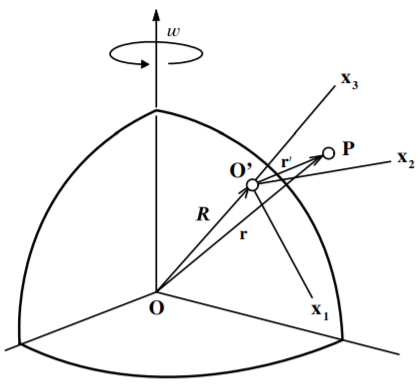$$\require{cancel}$$

# 12.10: Effective gravitational force near the surface of the Earth

$$\newcommand{\vecs}{\overset { \rightharpoonup} {\mathbf{#1}} }$$ $$\newcommand{\vecd}{\overset{-\!-\!\rightharpoonup}{\vphantom{a}\smash {#1}}}$$$$\newcommand{\id}{\mathrm{id}}$$ $$\newcommand{\Span}{\mathrm{span}}$$ $$\newcommand{\kernel}{\mathrm{null}\,}$$ $$\newcommand{\range}{\mathrm{range}\,}$$ $$\newcommand{\RealPart}{\mathrm{Re}}$$ $$\newcommand{\ImaginaryPart}{\mathrm{Im}}$$ $$\newcommand{\Argument}{\mathrm{Arg}}$$ $$\newcommand{\norm}{\| #1 \|}$$ $$\newcommand{\inner}{\langle #1, #2 \rangle}$$ $$\newcommand{\Span}{\mathrm{span}}$$ $$\newcommand{\id}{\mathrm{id}}$$ $$\newcommand{\Span}{\mathrm{span}}$$ $$\newcommand{\kernel}{\mathrm{null}\,}$$ $$\newcommand{\range}{\mathrm{range}\,}$$ $$\newcommand{\RealPart}{\mathrm{Re}}$$ $$\newcommand{\ImaginaryPart}{\mathrm{Im}}$$ $$\newcommand{\Argument}{\mathrm{Arg}}$$ $$\newcommand{\norm}{\| #1 \|}$$ $$\newcommand{\inner}{\langle #1, #2 \rangle}$$ $$\newcommand{\Span}{\mathrm{span}}$$

Consider that the translational acceleration of the center of the Earth can be neglected, and thus a set of non-rotating axes through the center of the Earth can be assumed to be approximately an inertial frame. The effects of the motion of the Earth around the Sun, or the motion of the Solar system in our Galaxy, are small compared with the effects due to the rotation of the Earth.Figure $$\PageIndex{1}$$: Rotating frame at the surface of the Earth.

Consider a rotating frame attached to the surface of the earth as shown in Figure $$\PageIndex{1}$$. The vector with respect to the center of the Earth $$\mathbf{r}$$ can be decomposed into a vector to the origin of the reference frame fixed to the surface of the Earth $$\mathbf{R}$$, plus the vector with respect to this surface reference frame $$\mathbf{r}^{\prime}$$.

$\mathbf{r} = \mathbf{R} + \mathbf{r}^{\prime}$

If the external force is separated into the gravitational term $$m\mathbf{g}$$, plus some other physical force $$\mathbf{F}$$, then the acceleration in the non-inertial surface frame of reference is

$\mathbf{a}^{\prime} = \frac{\mathbf{F}}{m} +\mathbf{g}−(\mathbf{A} + 2\boldsymbol{\omega} \times \mathbf{v}^{\prime} + \boldsymbol{\omega} \times (\boldsymbol{\omega} \times \mathbf{r}^{\prime} ) + \boldsymbol{\dot{\omega}} \times \mathbf{r}^{\prime} )$

But

$\mathbf{V} = \left(\frac{d\mathbf{R}}{dt}\right)_{fixed} = \left(\frac{d\mathbf{R}}{dt}\right)_{rotating} + \boldsymbol{\omega} \times \mathbf{R} = \boldsymbol{\omega} \times \mathbf{R}$

since in the rotating frame $$\left(\frac{d\mathbf{R}}{dt}\right)_{rotating} = 0$$. Also the acceleration

$\mathbf{A} = \left(\frac{d\mathbf{V}}{dt}\right)_{fixed} = \left(\frac{d\mathbf{V}}{dt}\right)_{rotating} + \boldsymbol{\omega} \times \mathbf{V} = \boldsymbol{\omega} \times (\boldsymbol{\omega} \times \mathbf{R})$

since $$\left(\frac{d\mathbf{V}}{dt}\right)_{rotating} = 0$$. Substituting this into the above equation gives

\begin{aligned} \mathbf{a}^{\prime} & = & \frac{\mathbf{F}}{m} + \mathbf{g} − (2\boldsymbol{\omega} \times \mathbf{v}^{\prime} + \boldsymbol{\omega} \times (\boldsymbol{\omega} \times [\mathbf{r}^{\prime} + \mathbf{R}]) + \boldsymbol{\dot{\omega}} \times \mathbf{r}^{\prime} ) \\ & = & \frac{\mathbf{F}}{m} + \mathbf{g} − (2\boldsymbol{\omega} \times \mathbf{v}^{\prime} + \boldsymbol{\omega} \times (\boldsymbol{\omega} \times \mathbf{r}) + \boldsymbol{\dot{\omega}} \times \mathbf{r}^{\prime} ) \end{aligned}

where $$\mathbf{r}$$ is with respect to the center of the Earth. This is as expected directly from equation $$(12.6.8)$$. Since the angular frequency of the earth is a constant then $$\dot{\omega} \times \mathbf{r}^{\prime} = 0$$. Thus the acceleration can be written as

$\mathbf{a}^{\prime} = \frac{\mathbf{F}}{m} + [\mathbf{g} − \boldsymbol{\omega} \times (\boldsymbol{\omega} \times \mathbf{ r})] − 2\boldsymbol{\omega} \times \mathbf{v}^{\prime} \label{12.52}$

The term in the square brackets combines the gravitational acceleration plus the centrifugal acceleration.

A measurement of the Earth’s gravitational acceleration actually measures the term in the square brackets in Equation \ref{12.52}, that is, an effective gravitational acceleration where

$\mathbf{g}_{eff} = \mathbf{g} − \boldsymbol{\omega} \times (\boldsymbol{\omega} \times \mathbf{ r})$

near the surface of the earth $$\mathbf{r} \approx \mathbf{R}$$. The effective gravitational force does not point towards the center of the Earth as shown in Figure $$\PageIndex{2}$$. A plumb line points, or an object falls, in the direction of $$\mathbf{g}_{eff}$$. The shape of the earth is such that the Earth’s surface is perpendicular to $$\mathbf{g}_{eff}$$. This is the reason why the earth is distorted into an oblate ellipsoid, that is, it is flattened at the poles.Figure $$\PageIndex{2}$$: Effective gravitational acceleration.

The angle $$\alpha$$ between $$\mathbf{g}_{eff}$$ and the line pointing to the center of the earth is dependent on the latitude $$\lambda = \frac{\pi}{2} −\theta$$. Note that the colatitude $$\theta$$ is taken to be zero at the North pole whereas the latitude $$\lambda$$ is taken to be zero at the equator. The angle $$\alpha$$ can be estimated by assuming that $$r^{\prime} << R$$, then the centrifugal term then can be approximated by

$|\boldsymbol{\omega} \times (\boldsymbol{\omega} \times \mathbf{ r})| \approx \omega^2 R \sin \theta = \omega^2 R \cos \lambda$

This is quite small for the Earth since $$\omega = 0.73 \times 10^{−4}$$ $$rads/s$$ and $$R = 6371$$ $$km$$, leading to a correction term $$\omega^2 R \cos \lambda = 0.03 \cos \lambda$$ $$m/s^2$$. Since

$g^{horizontal}_{eff} = \omega^2 R \cos \lambda \sin \lambda$

and

$g^{vertical}_{eff} = g − \omega^2 R \cos^2 \lambda$

Then the angle $$\alpha$$ between $$\mathbf{g}_{eff}$$ and $$\mathbf{g}$$ is given by

$\alpha \simeq \tan \alpha = \frac{g^{horizontal}_{eff}}{g^{vertical}_{eff}} = \frac{\omega^2 R \cos \lambda \sin \lambda}{g − \omega^2 R \cos^2 \lambda}$

This has a maximum value at $$\lambda = 45^{\circ}$$ which is $$\alpha = 0.0088^{\circ}$$.

This page titled 12.10: Effective gravitational force near the surface of the Earth is shared under a CC BY-NC-SA 4.0 license and was authored, remixed, and/or curated by Douglas Cline via source content that was edited to the style and standards of the LibreTexts platform; a detailed edit history is available upon request.